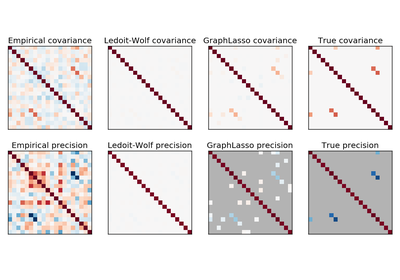# `sklearn.covariance`.ledoit_wolf¶

`sklearn.covariance.``ledoit_wolf`(X, assume_centered=False, block_size=1000)[source]

Estimates the shrunk Ledoit-Wolf covariance matrix.

Read more in the User Guide.

Parameters: X : array-like, shape (n_samples, n_features) Data from which to compute the covariance estimate assume_centered : boolean, default=False If True, data are not centered before computation. Useful to work with data whose mean is significantly equal to zero but is not exactly zero. If False, data are centered before computation. block_size : int, default=1000 Size of the blocks into which the covariance matrix will be split. This is purely a memory optimization and does not affect results. shrunk_cov : array-like, shape (n_features, n_features) Shrunk covariance. shrinkage : float Coefficient in the convex combination used for the computation of the shrunk estimate.

Notes

The regularized (shrunk) covariance is:

(1 - shrinkage)*cov
• shrinkage * mu * np.identity(n_features)

where mu = trace(cov) / n_features

## Examples using `sklearn.covariance.ledoit_wolf`¶Sparse inverse covariance estimation